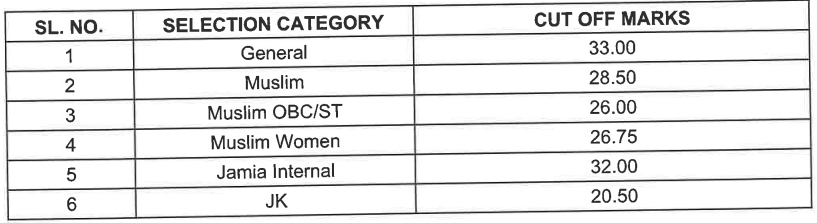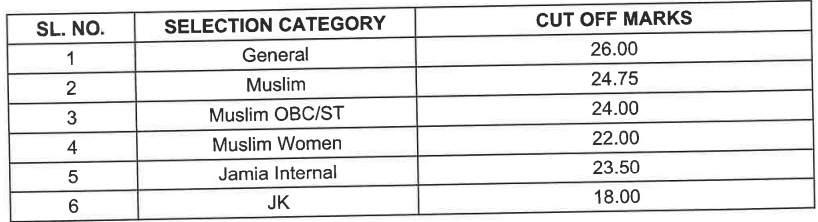# Jamia B.Sc (Hons) Mathematics Previous 4 Years Entrance Test Papers | 2022 and Before

## Jamia B.Sc (Hons) Mathematics Previous 4 Years Entrance Test Papers | 2022 and Before

B.Sc. (Hons) in Mathematics focuses on the in-depth study of a number of mathematical disciplines, including algebra, geometry, analysis, and statistics. The normal length of the programme is three years, and it provides a challenging and thorough curriculum in mathematics.

A strong foundation in mathematical theory and problem-solving abilities is what the B.Sc. (Hons.) Mathematics curriculum aims to give students. Many subjects, including calculus, linear algebra, number theory, discrete mathematics, probability theory, and mathematical modelling are covered in the programme.

The programme stresses practical applications of mathematics in addition to academic study. To get practical experience in the subject, students may be given the chance to work on research projects, attend seminars, and take part in internships.

Graduates of the programme can go on to work in a range of industries, including data analysis, finance, engineering, teaching, and research. Graduates of the B.Sc. (Hons) Mathematics programme occasionally go on to get postgraduate degrees in mathematics or related disciplines.

In conclusion, the B.Sc. (Hons) Mathematics programme is a demanding and specialised undergraduate course of study that gives students a solid foundation in both mathematical theory and applications. Graduates are prepared for positions in a variety of fields where problem-solving and quantitative skills are highly appreciated.

### Entrance Exam Details

Maximum Marks: 100

Total Questions: 100

Time: 90 minutes

Negative Marking: Yes, 0.25 marks deduction for every wrong answer.

Total Seats: 90

Course Fee: ₹7,800 per year.

### Cut-Off (2022):

B.Sc.(Hons) (Mathematics)B.Sc.(Hons) (Applied Mathematics)VIEW PDF

VIEW PDF

VIEW PDF

### Jamia B.Sc.(Hons) Mathematics Entrance/Admission Test Paper, 2019:

VIEW PDF